For other uses, see Addition (disambiguation).3 + 2 = 5 with apples, a popular choice in textbooks

Addition (often signified by the plus symbol "+") is one of the four basic operations of arithmetic, with the others being subtraction, multiplication and division. The addition of two whole numbers is the total amount of those quantities combined. For example, in the picture on the right, there is a combination of three apples and two apples together, making a total of five apples. This observation is equivalent to the mathematical expression "3 + 2 = 5" i.e., "3 add 2 is equal to 5".

Besides counting fruits, addition can also represent combining other physical objects. Using systematic generalizations, addition can also be defined on more abstract quantities, such as integers, rational numbers, real numbers and complex numbers and other abstract objects such as vectors and matrices.

In arithmetic, rules for addition involving fractions and negative numbers have been devised amongst others. In algebra, addition is studied more abstractly.

Addition has several important properties. It is commutative, meaning that order does not matter, and it is associative, meaning that when one adds more than two numbers, the order in which addition is performed does not matter (see Summation). Repeated addition of 1 is the same as counting; addition of 0 does not change a number. Addition also obeys predictable rules concerning related operations such as subtraction and multiplication.

Performing addition is one of the simplest numerical tasks. Addition of very small numbers is accessible to toddlers; the most basic task, 1 + 1, can be performed by infants as young as five months and even some non-human animals. In primary education, students are taught to add numbers in the decimal system, starting with single digits and progressively tackling more difficult problems. Mechanical aids range from the ancient abacus to the modern computer, where research on the most efficient implementations of addition continues to this day.

## Notation and terminologyThe plus sign

Addition is written using the plus sign "+" between the terms; that is, in infix notation. The result is expressed with an equals sign. For example,("one plus one equals two")("two plus two equals four")("three plus three equals six")(see "associativity" below)(see "multiplication" below)

There are also situations where addition is "understood" even though no symbol appears:5 + 12 = 17
• A column of numbers, with the last number in the column underlined, usually indicates that the numbers in the column are to be added, with the sum written below the underlined number.
• A whole number followed immediately by a fraction indicates the sum of the two, called a mixed number. For example,
3½ = 3 + ½ = 3.5.
This notation can cause confusion since in most other contexts juxtaposition denotes multiplication instead.

The sum of a series of related numbers can be expressed through capital sigma notation, which compactly denotes iteration. For example,The numbers or the objects to be added in general addition are collectively referred to as the terms, the addends or the summands; this terminology carries over to the summation of multiple terms. This is to be distinguished from factors, which are multiplied. Some authors call the first addend the augend. In fact, during the Renaissance, many authors did not consider the first addend an "addend" at all. Today, due to the commutative property of addition, "augend" is rarely used, and both terms are generally called addends.

All of the above terminology derives from Latin. "Addition" and "add" are English words derived from the Latin verb addere, which is in turn a compound of ad "to" and dare "to give", from the Proto-Indo-European root *deh₃- "to give"; thus to add is to give to. Using the gerundive suffix -nd results in "addend", "thing to be added". Likewise from augere "to increase", one gets "augend", "thing to be increased".Redrawn illustration from The Art of Nombryng, one of the first English arithmetic texts, in the 15th century

"Sum" and "summand" derive from the Latin noun summa "the highest, the top" and associated verb summare. This is appropriate not only because the sum of two positive numbers is greater than either, but because it was common for the ancient Greeks and Romans to add upward, contrary to the modern practice of adding downward, so that a sum was literally higher than the addends. Addere and summare date back at least to Boethius, if not to earlier Roman writers such as Vitruvius and Frontinus; Boethius also used several other terms for the addition operation. The later Middle English terms "adden" and "adding" were popularized by Chaucer.

The plus sign "+" (Unicode:U+002B; ASCII: &#43;) is an abbreviation of the Latin word et, meaning "and". It appears in mathematical works dating back to at least 1489.

## Interpretations

Addition is used to model countless physical processes. Even for the simple case of adding natural numbers, there are many possible interpretations and even more visual representations.

### Combining sets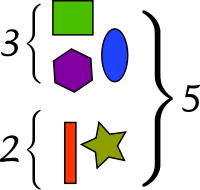Possibly the most fundamental interpretation of addition lies in combining sets:

• When two or more disjoint collections are combined into a single collection, the number of objects in the single collection is the sum of the number of objects in the original collections.

This interpretation is easy to visualize, with little danger of ambiguity. It is also useful in higher mathematics; for the rigorous definition it inspires, see Natural numbers below. However, it is not obvious how one should extend this version of addition to include fractional numbers or negative numbers.

One possible fix is to consider collections of objects that can be easily divided, such as pies or, still better, segmented rods. Rather than just combining collections of segments, rods can be joined end-to-end, which illustrates another conception of addition: adding not the rods but the lengths of the rods.

### Extending a lengthA second interpretation of addition comes from extending an initial length by a given length:

• When an original length is extended by a given amount, the final length is the sum of the original length and the length of the extension.

The sum a + b can be interpreted as a binary operation that combines a and b, in an algebraic sense, or it can be interpreted as the addition of b more units to a. Under the latter interpretation, the parts of a sum a + b play asymmetric roles, and the operation a + b is viewed as applying the unary operation +b to a. Instead of calling both a and b addends, it is more appropriate to call a the augend in this case, since a plays a passive role. The unary view is also useful when discussing subtraction, because each unary addition operation has an inverse unary subtraction operation, and vice versa.

## Properties

### Commutativity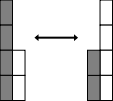4 + 2 = 2 + 4 with blocks

Addition is commutative: one can change the order of the terms in a sum, and the result is the same. Symbolically, if a and b are any two numbers, then

a + b = b + a.

The fact that addition is commutative is known as the "commutative law of addition". This phrase suggests that there are other commutative laws: for example, there is a commutative law of multiplication. However, many binary operations are not commutative, such as subtraction and division, so it is misleading to speak of an unqualified "commutative law".

### Associativity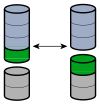2+(1+3) = (2+1)+3 with segmented rods

Addition is associative: when adding three or more numbers, the order of operations does not matter .

As an example, should the expression a + b + c be defined to mean (a + b) + c or a + (b + c)? That addition is associative tells us that the choice of definition is irrelevant. For any three numbers a, b, and c, it is true that (a + b) + c = a + (b + c). For example, (1 + 2) + 3 = 3 + 3 = 6 = 1 + 5 = 1 + (2 + 3).

When addition is used together with other operations, the order of operations becomes important. In the standard order of operations, addition is a lower priority than exponentiation, nth roots, multiplication and division, but is given equal priority to subtraction.

### Identity element5 + 0 = 5 with bags of dots

When adding zero to any number, the quantity does not change; zero is the identity element for addition, also known as the additive identity. In symbols, for any a,

a + 0 = 0 + a = a.

This law was first identified in Brahmagupta's Brahmasphutasiddhanta in 628 AD, although he wrote it as three separate laws, depending on whether a is negative, positive, or zero itself, and he used words rather than algebraic symbols. Later Indian mathematicians refined the concept; around the year 830, Mahavira wrote, "zero becomes the same as what is added to it", corresponding to the unary statement 0 + a = a. In the 12th century, Bhaskara wrote, "In the addition of cipher, or subtraction of it, the quantity, positive or negative, remains the same", corresponding to the unary statement a + 0 = a.

### Successor

In the context of integers, addition of one also plays a special role: for any integer a, the integer (a + 1) is the least integer greater than a, also known as the successor of a. For instance, 3 is the successor of 2 and 7 is the successor of 6. Because of this succession, the value of a + b can also be seen as thesuccessor of a, making addition iterated succession. For examples, 6 + 2 is 8, because 8 is the successor of 7, which is the successor of 6, making 8 the 2nd successor of 6.

### Units

To numerically add physical quantities with units, they must be expressed with common units. For example, adding 50 millilitres to 150 millilitres gives 200 millilitres. However, if a measure of 5 feet is extended by 2 inches, the sum is 62 inches, since 60 inches is synonymous with 5 feet. On the other hand, it is usually meaningless to try to add 3 meters and 4 square meters, since those units are incomparable; this sort of consideration is fundamental in dimensional analysis.

### Innate ability

Studies on mathematical development starting around the 1980s have exploited the phenomenon of habituation: infants look longer at situations that are unexpected. A seminal experiment by Karen Wynn in 1992 involving Mickey Mouse dolls manipulated behind a screen demonstrated that five-month-old infants expect 1 + 1 to be 2, and they are comparatively surprised when a physical situation seems to imply that 1 + 1 is either 1 or 3. This finding has since been affirmed by a variety of laboratories using different methodologies. Another 1992 experiment with older toddlers, between 18 and 35 months, exploited their development of motor control by allowing them to retrieve ping-pong balls from a box; the youngest responded well for small numbers, while older subjects were able to compute sums up to 5.

Even some nonhuman animals show a limited ability to add, particularly primates. In a 1995 experiment imitating Wynn's 1992 result (but using eggplants instead of dolls), rhesus macaque and cottontop tamarin monkeys performed similarly to human infants. More dramatically, after being taught the meanings of the Arabic numerals 0 through 4, one chimpanzee was able to compute the sum of two numerals without further training. More recently, Asian elephants have demonstrated an ability to perform basic arithmetic.

Typically, children first master counting. When given a problem that requires that two items and three items be combined, young children model the situation with physical objects, often fingers or a drawing, and then count the total. As they gain experience, they learn or discover the strategy of "counting-on": asked to find two plus three, children count three past two, saying "three, four, five" (usually ticking off fingers), and arriving at five. This strategy seems almost universal; children can easily pick it up from peers or teachers. Most discover it independently. With additional experience, children learn to add more quickly by exploiting the commutativity of addition by counting up from the larger number, in this case starting with three and counting "four, five." Eventually children begin to recall certain addition facts ("number bonds"), either through experience or rote memorization. Once some facts are committed to memory, children begin to derive unknown facts from known ones. For example, a child asked to add six and seven may know that 6 + 6 = 12 and then reason that 6 + 7 is one more, or 13. Such derived facts can be found very quickly and most elementary school students eventually rely on a mixture of memorized and derived facts to add fluently.

Different nations introduce whole numbers and arithmetic at different ages, with many countries teaching addition in pre-school. However, throughout the world, addition is taught by the end of the first year of elementary school.

Children are often presented with the addition table of pairs of numbers from 1 to 10 to memorize. Knowing this, one can perform any addition.

 1 + 0 = 1 1 + 1 = 2 1 + 2 = 3 1 + 3 = 4 1 + 4 = 5 1 + 5 = 6 1 + 6 = 7 1 + 7 = 8 1 + 8 = 9 1 + 9 = 10 1 + 10 = 11
 2 + 0 = 2 2 + 1 = 3 2 + 2 = 4 2 + 3 = 5 2 + 4 = 6 2 + 5 = 7 2 + 6 = 8 2 + 7 = 9 2 + 8 = 10 2 + 9 = 11 2 + 10 = 12
 3 + 0 = 3 3 + 1 = 4 3 + 2 = 5 3 + 3 = 6 3 + 4 = 7 3 + 5 = 8 3 + 6 = 9 3 + 7 = 10 3 + 8 = 11 3 + 9 = 12 3 + 10 = 13
 4 + 0 = 4 4 + 1 = 5 4 + 2 = 6 4 + 3 = 7 4 + 4 = 8 4 + 5 = 9 4 + 6 = 10 4 + 7 = 11 4 + 8 = 12 4 + 9 = 13 4 + 10 = 14
 5 + 0 = 5 5 + 1 = 6 5 + 2 = 7 5 + 3 = 8 5 + 4 = 9 5 + 5 = 10 5 + 6 = 11 5 + 7 = 12 5 + 8 = 13 5 + 9 = 14 5 + 10 = 15
 6 + 0 = 6 6 + 1 = 7 6 + 2 = 8 6 + 3 = 9 6 + 4 = 10 6 + 5 = 11 6 + 6 = 12 6 + 7 = 13 6 + 8 = 14 6 + 9 = 15 6 + 10 = 16
 7 + 0 = 7 7 + 1 = 8 7 + 2 = 9 7 + 3 = 10 7 + 4 = 11 7 + 5 = 12 7 + 6 = 13 7 + 7 = 14 7 + 8 = 15 7 + 9 = 16 7 + 10 = 17
 8 + 0 = 8 8 + 1 = 9 8 + 2 = 10 8 + 3 = 11 8 + 4 = 12 8 + 5 = 13 8 + 6 = 14 8 + 7 = 15 8 + 8 = 16 8 + 9 = 17 8 + 10 = 18
 9 + 0 = 9 9 + 1 = 10 9 + 2 = 11 9 + 3 = 12 9 + 4 = 13 9 + 5 = 14 9 + 6 = 15 9 + 7 = 16 9 + 8 = 17 9 + 9 = 18 9 + 10 = 19
 10 + 0 = 10 10 + 1 = 11 10 + 2 = 12 10 + 3 = 13 10 + 4 = 14 10 + 5 = 15 10 + 6 = 16 10 + 7 = 17 10 + 8 = 18 10 + 9 = 19 10 + 10 = 20

### Decimal system

The prerequisite to addition in the decimal system is the fluent recall or derivation of the 100 single-digit "addition facts". One could memorize all the facts by rote, but pattern-based strategies are more enlightening and, for most people, more efficient:

• Commutative property: Mentioned above, using the pattern a + b = b + a reduces the number of "addition facts" from 100 to 55.
• One or two more: Adding 1 or 2 is a basic task, and it can be accomplished through counting on or, ultimately, intuition.
• Zero: Since zero is the additive identity, adding zero is trivial. Nonetheless, in the teaching of arithmetic, some students are introduced to addition as a process that always increases the addends; word problems may help rationalize the "exception" of zero.
• Doubles: Adding a number to itself is related to counting by two and to multiplication. Doubles facts form a backbone for many related facts, and students find them relatively easy to grasp.
• Near-doubles: Sums such as 6 + 7 = 13 can be quickly derived from the doubles fact 6 + 6 = 12 by adding one more, or from 7 + 7 = 14 but subtracting one.
• Five and ten: Sums of the form 5 + x and 10 + x are usually memorized early and can be used for deriving other facts. For example, 6 + 7 = 13 can be derived from 5 + 7 = 12 by adding one more.
• Making ten: An advanced strategy uses 10 as an intermediate for sums involving 8 or 9; for example, 8 + 6 = 8 + 2 + 4 = 10 + 4 = 14.

As students grow older, they commit more facts to memory, and learn to derive other facts rapidly and fluently. Many students never commit all the facts to memory, but can still find any basic fact quickly.

#### Carry

The standard algorithm for adding multidigit numbers is to align the addends vertically and add the columns, starting from the ones column on the right. If a column exceeds ten, the extra digit is "carried" into the next column. For example, in the addition 27 + 59

  ¹
27
+ 59
————
86

7 + 9 = 16, and the digit 1 is the carry. An alternate strategy starts adding from the most significant digit on the left; this route makes carrying a little clumsier, but it is faster at getting a rough estimate of the sum. There are many alternative methods.

Decimal fractions can be added by a simple modification of the above process. One aligns two decimal fractions above each other, with the decimal point in the same location. If necessary, one can add trailing zeros to a shorter decimal to make it the same length as the longer decimal. Finally, one performs the same addition process as above, except the decimal point is placed in the answer, exactly where it was placed in the summands.

As an example, 45.1 + 4.34 can be solved as follows:

   4 5 . 1 0
+  0 4 . 3 4
————————————
4 9 . 4 4

#### Scientific notation

In scientific notation, numbers are written in the form, whereis the significand andis the exponential part. Addition requires two numbers in scientific notation to be represented using the same exponential part, so that the significand can be simply added or subtracted.

For example:Addition in other bases is very similar to decimal addition. As an example, one can consider addition in binary. Adding two single-digit binary numbers is relatively simple, using a form of carrying:

0 + 0 → 0
0 + 1 → 1
1 + 0 → 1
1 + 1 → 0, carry 1 (since 1 + 1 = 2 = 0 + (1 × 21) )

Adding two "1" digits produces a digit "0", while 1 must be added to the next column. This is similar to what happens in decimal when certain single-digit numbers are added together; if the result equals or exceeds the value of the radix (10), the digit to the left is incremented:

5 + 5 → 0, carry 1 (since 5 + 5 = 10 = 0 + (1 × 101) )
7 + 9 → 6, carry 1 (since 7 + 9 = 16 = 6 + (1 × 101) )

This is known as carrying. When the result of an addition exceeds the value of a digit, the procedure is to "carry" the excess amount divided by the radix (that is, 10/10) to the left, adding it to the next positional value. This is correct since the next position has a weight that is higher by a factor equal to the radix. Carrying works the same way in binary:

  1 1 1 1 1    (carried digits)
0 1 1 0 1
+   1 0 1 1 1
—————————————
1 0 0 1 0 0 = 36

In this example, two numerals are being added together: 011012 (1310) and 101112 (2310). The top row shows the carry bits used. Starting in the rightmost column, 1 + 1 = 102. The 1 is carried to the left, and the 0 is written at the bottom of the rightmost column. The second column from the right is added: 1 + 0 + 1 = 102 again; the 1 is carried, and 0 is written at the bottom. The third column: 1 + 1 + 1 = 112. This time, a 1 is carried, and a 1 is written in the bottom row. Proceeding like this gives the final answer 1001002 (36 decimal).

### Computers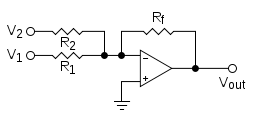Analog computers work directly with physical quantities, so their addition mechanisms depend on the form of the addends. A mechanical adder might represent two addends as the positions of sliding blocks, in which case they can be added with an averaging lever. If the addends are the rotation speeds of two shafts, they can be added with a differential. A hydraulic adder can add the pressures in two chambers by exploiting Newton's second law to balance forces on an assembly of pistons. The most common situation for a general-purpose analog computer is to add two voltages (referenced to ground); this can be accomplished roughly with a resistor network, but a better design exploits an operational amplifier.

Addition is also fundamental to the operation of digital computers, where the efficiency of addition, in particular the carry mechanism, is an important limitation to overall performance.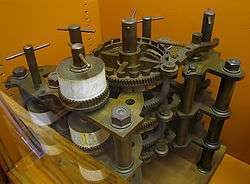Part of Charles Babbage's Difference Engine including the addition and carry mechanisms

The abacus, also called a counting frame, is a calculating tool that was in use centuries before the adoption of the written modern numeral system and is still widely used by merchants, traders and clerks in Asia, Africa, and elsewhere; it dates back to at least 2700–2300 BC, when it was used in Sumer.

Blaise Pascal invented the mechanical calculator in 1642; it was the first operational adding machine. It made use of a gravity-assisted carry mechanism. It was the only operational mechanical calculator in the 17th century and the earliest automatic, digital computers. Pascal's calculator was limited by its carry mechanism, which forced its wheels to only turn one way so it could add. To subtract, the operator had to use the Pascal's calculator's complement, which required as many steps as an addition. Giovanni Poleni followed Pascal, building the second functional mechanical calculator in 1709, a calculating clock made of wood that, once setup, could multiply two numbers automatically.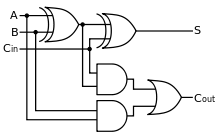"Full adder" logic circuit that adds two binary digits, A and B, along with a carry input Cin, producing the sum bit, S, and a carry output, Cout.

Adders execute integer addition in electronic digital computers, usually using binary arithmetic. The simplest architecture is the ripple carry adder, which follows the standard multi-digit algorithm. One slight improvement is the carry skip design, again following human intuition; one does not perform all the carries in computing 999 + 1, but one bypasses the group of 9s and skips to the answer.

// Iterative Algorithm
int carry = 0;
while (y != 0){
carry = AND(x, y);   // Logical AND
x     = XOR(x, y);   // Logical XOR
y     = carry << 1;  // left bitshift carry by one
}
return x;
}
// Recursive Algorithm
return x if (y == 0) else add(XOR(x, y) , AND(x, y) << 1);
}


On a computer, if the result of an addition is too large to store, an arithmetic overflow occurs, resulting in an incorrect answer. Unanticipated arithmetic overflow is a fairly common cause of program errors. Such overflow bugs may be hard to discover and diagnose because they may manifest themselves only for very large input data sets, which are less likely to be used in validation tests. One especially notable such error was the Y2K bug, where overflow errors due to using a 2-digit format for years caused significant computer problems in 2000.

To prove the usual properties of addition, one must first define addition for the context in question. Addition is first defined on the natural numbers. In set theory, addition is then extended to progressively larger sets that include the natural numbers: the integers, the rational numbers, and the real numbers. (In mathematics education, positive fractions are added before negative numbers are even considered; this is also the historical route.)

### Natural numbers

Further information: Natural number

There are two popular ways to define the sum of two natural numbers a and b. If one defines natural numbers to be the cardinalities of finite sets, (the cardinality of a set is the number of elements in the set), then it is appropriate to define their sum as follows:

• Let N(S) be the cardinality of a set S. Take two disjoint sets A and B, with N(A) = a and N(B) = b. Then a + b is defined as.

Here, A U B is the union of A and B. An alternate version of this definition allows A and B to possibly overlap and then takes their disjoint union, a mechanism that allows common elements to be separated out and therefore counted twice.

The other popular definition is recursive:

• Let n+ be the successor of n, that is the number following n in the natural numbers, so 0+=1, 1+=2. Define a + 0 = a. Define the general sum recursively by a + (b+) = (a + b)+. Hence 1 + 1 = 1 + 0+ = (1 + 0)+ = 1+ = 2.

Again, there are minor variations upon this definition in the literature. Taken literally, the above definition is an application of the Recursion Theorem on the partially ordered set N2. On the other hand, some sources prefer to use a restricted Recursion Theorem that applies only to the set of natural numbers. One then considers a to be temporarily "fixed", applies recursion on b to define a function "a + ", and pastes these unary operations for all a together to form the full binary operation.

This recursive formulation of addition was developed by Dedekind as early as 1854, and he would expand upon it in the following decades. He proved the associative and commutative properties, among others, through mathematical induction.

### Integers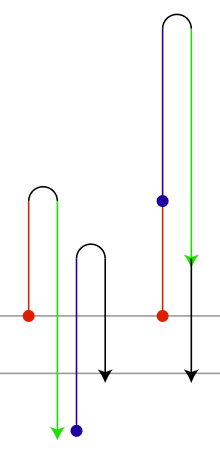Defining (−2) + 1 using only addition of positive numbers: (2 − 4) + (3 − 2) = 5 − 6.
Further information: Integer

The simplest conception of an integer is that it consists of an absolute value (which is a natural number) and a sign (generally either positive or negative). The integer zero is a special third case, being neither positive nor negative. The corresponding definition of addition must proceed by cases:

• For an integer n, let |n| be its absolute value. Let a and b be integers. If either a or b is zero, treat it as an identity. If a and b are both positive, define a + b = |a| + |b|. If a and b are both negative, define a + b = −(|a|+|b|). If a and b have different signs, define a + b to be the difference between |a| and |b|, with the sign of the term whose absolute value is larger. As an example, -6 + 4 = -2; because -6 and 4 have different signs, their absolute values are subtracted, and since the negative term is larger, the answer is negative.

Although this definition can be useful for concrete problems, it is far too complicated to produce elegant general proofs; there are too many cases to consider.

A much more convenient conception of the integers is the Grothendieck group construction. The essential observation is that every integer can be expressed (not uniquely) as the difference of two natural numbers, so we may as well define an integer as the difference of two natural numbers. Addition is then defined to be compatible with subtraction:

• Given two integers ab and cd, where a, b, c, and d are natural numbers, define (ab) + (cd) = (a + c) − (b + d).

### Rational numbers (fractions)

Addition of rational numbers can be computed using the least common denominator, but a conceptually simpler definition involves only integer addition and multiplication:

• DefineAs an example, the sum.

Addition of fractions is much simpler when the denominators are the same; in this case, one can simply add the numerators while leaving the denominator the same:, so.

The commutativity and associativity of rational addition is an easy consequence of the laws of integer arithmetic. For a more rigorous and general discussion, see field of fractions.

### Real numbers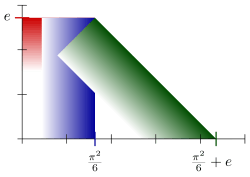Adding π2/6 and e using Dedekind cuts of rationals

A common construction of the set of real numbers is the Dedekind completion of the set of rational numbers. A real number is defined to be a Dedekind cut of rationals: a non-empty set of rationals that is closed downward and has no greatest element. The sum of real numbers a and b is defined element by element:

• DefineThis definition was first published, in a slightly modified form, by Richard Dedekind in 1872. The commutativity and associativity of real addition are immediate; defining the real number 0 to be the set of negative rationals, it is easily seen to be the additive identity. Probably the trickiest part of this construction pertaining to addition is the definition of additive inverses.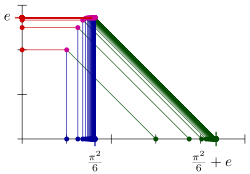Adding π2/6 and e using Cauchy sequences of rationals

Unfortunately, dealing with multiplication of Dedekind cuts is a time-consuming case-by-case process similar to the addition of signed integers. Another approach is the metric completion of the rational numbers. A real number is essentially defined to be the a limit of a Cauchy sequence of rationals, lim an. Addition is defined term by term:

• DefineThis definition was first published by Georg Cantor, also in 1872, although his formalism was slightly different. One must prove that this operation is well-defined, dealing with co-Cauchy sequences. Once that task is done, all the properties of real addition follow immediately from the properties of rational numbers. Furthermore, the other arithmetic operations, including multiplication, have straightforward, analogous definitions.

### Complex numbers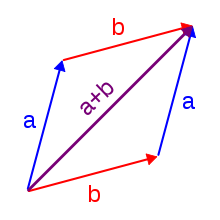Addition of two complex numbers can be done geometrically by constructing a parallelogram.

Complex numbers are added by adding the real and imaginary parts of the summands. That is to say:Using the visualization of complex numbers in the complex plane, the addition has the following geometric interpretation: the sum of two complex numbers A and B, interpreted as points of the complex plane, is the point X obtained by building a parallelogram three of whose vertices are O, A and B. Equivalently, X is the point such that the triangles with vertices O, A, B, and X, B, A, are congruent.

## Generalizations

There are many binary operations that can be viewed as generalizations of the addition operation on the real numbers. The field of abstract algebra is centrally concerned with such generalized operations, and they also appear in set theory and category theory.

In linear algebra, a vector space is an algebraic structure that allows for adding any two vectors and for scaling vectors. A familiar vector space is the set of all ordered pairs of real numbers; the ordered pair (a,b) is interpreted as a vector from the origin in the Euclidean plane to the point (a,b) in the plane. The sum of two vectors is obtained by adding their individual coordinates:

(a,b) + (c,d) = (a+c,b+d).

This addition operation is central to classical mechanics, in which vectors are interpreted as forces.

Matrix addition is defined for two matrices of the same dimensions. The sum of two m × n (pronounced "m by n") matrices A and B, denoted by A + B, is again an m × n matrix computed by adding corresponding elements:For example:#### Modular arithmetic

Main article: Modular arithmetic

In modular arithmetic, the set of integers modulo 12 has twelve elements; it inherits an addition operation from the integers that is central to musical set theory. The set of integers modulo 2 has just two elements; the addition operation it inherits is known in Boolean logic as the "exclusive or" function. In geometry, the sum of two angle measures is often taken to be their sum as real numbers modulo 2π. This amounts to an addition operation on the circle, which in turn generalizes to addition operations on many-dimensional tori.

The general theory of abstract algebra allows an "addition" operation to be any associative and commutative operation on a set. Basic algebraic structures with such an addition operation include commutative monoids and abelian groups.

### Addition in set theory and category theory

A far-reaching generalization of addition of natural numbers is the addition of ordinal numbers and cardinal numbers in set theory. These give two different generalizations of addition of natural numbers to the transfinite. Unlike most addition operations, addition of ordinal numbers is not commutative. Addition of cardinal numbers, however, is a commutative operation closely related to the disjoint union operation.

In category theory, disjoint union is seen as a particular case of the coproduct operation, and general coproducts are perhaps the most abstract of all the generalizations of addition. Some coproducts, such as Direct sum and Wedge sum, are named to evoke their connection with addition.

## Related operations

Addition, along with subtraction, multiplication and division, is considered one of the basic operations and is used in elementary arithmetic.

### Arithmetic

Subtraction can be thought of as a kind of addition—that is, the addition of an additive inverse. Subtraction is itself a sort of inverse to addition, in that adding x and subtracting x are inverse functions.

Given a set with an addition operation, one cannot always define a corresponding subtraction operation on that set; the set of natural numbers is a simple example. On the other hand, a subtraction operation uniquely determines an addition operation, an additive inverse operation, and an additive identity; for this reason, an additive group can be described as a set that is closed under subtraction.

Multiplication can be thought of as repeated addition. If a single term x appears in a sum n times, then the sum is the product of n and x. If n is not a natural number, the product may still make sense; for example, multiplication by −1 yields the additive inverse of a number.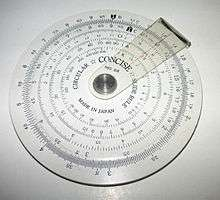A circular slide rule

In the real and complex numbers, addition and multiplication can be interchanged by the exponential function:

ea + b = ea eb.

This identity allows multiplication to be carried out by consulting a table of logarithms and computing addition by hand; it also enables multiplication on a slide rule. The formula is still a good first-order approximation in the broad context of Lie groups, where it relates multiplication of infinitesimal group elements with addition of vectors in the associated Lie algebra.

There are even more generalizations of multiplication than addition. In general, multiplication operations always distribute over addition; this requirement is formalized in the definition of a ring. In some contexts, such as the integers, distributivity over addition and the existence of a multiplicative identity is enough to uniquely determine the multiplication operation. The distributive property also provides information about addition; by expanding the product (1 + 1)(a + b) in both ways, one concludes that addition is forced to be commutative. For this reason, ring addition is commutative in general.

Division is an arithmetic operation remotely related to addition. Since a/b = a(b−1), division is right distributive over addition: (a + b) / c = a / c + b / c. However, division is not left distributive over addition; 1/ (2 + 2) is not the same as 1/2 + 1/2.

### Ordering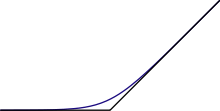Log-log plot of x + 1 and max (x, 1) from x = 0.001 to 1000

The maximum operation "max (a, b)" is a binary operation similar to addition. In fact, if two nonnegative numbers a and b are of different orders of magnitude, then their sum is approximately equal to their maximum. This approximation is extremely useful in the applications of mathematics, for example in truncating Taylor series. However, it presents a perpetual difficulty in numerical analysis, essentially since "max" is not invertible. If b is much greater than a, then a straightforward calculation of (a + b) − b can accumulate an unacceptable round-off error, perhaps even returning zero. See also Loss of significance.

The approximation becomes exact in a kind of infinite limit; if either a or b is an infinite cardinal number, their cardinal sum is exactly equal to the greater of the two. Accordingly, there is no subtraction operation for infinite cardinals.

Maximization is commutative and associative, like addition. Furthermore, since addition preserves the ordering of real numbers, addition distributes over "max" in the same way that multiplication distributes over addition:

a + max (b, c) = max (a + b, a + c).

For these reasons, in tropical geometry one replaces multiplication with addition and addition with maximization. In this context, addition is called "tropical multiplication", maximization is called "tropical addition", and the tropical "additive identity" is negative infinity. Some authors prefer to replace addition with minimization; then the additive identity is positive infinity.

Tying these observations together, tropical addition is approximately related to regular addition through the logarithm:

log (a + b) ≈ max (log a, log b),

which becomes more accurate as the base of the logarithm increases. The approximation can be made exact by extracting a constant h, named by analogy with Planck's constant from quantum mechanics, and taking the "classical limit" as h tends to zero:In this sense, the maximum operation is a dequantized version of addition.

Incrementation, also known as the successor operation, is the addition of 1 to a number.

Summation describes the addition of arbitrarily many numbers, usually more than just two. It includes the idea of the sum of a single number, which is itself, and the empty sum, which is zero. An infinite summation is a delicate procedure known as a series.

Counting a finite set is equivalent to summing 1 over the set.

Integration is a kind of "summation" over a continuum, or more precisely and generally, over a differentiable manifold. Integration over a zero-dimensional manifold reduces to summation.

Linear combinations combine multiplication and summation; they are sums in which each term has a multiplier, usually a real or complex number. Linear combinations are especially useful in contexts where straightforward addition would violate some normalization rule, such as mixing of strategies in game theory or superposition of states in quantum mechanics.

Convolution is used to add two independent random variables defined by distribution functions. Its usual definition combines integration, subtraction, and multiplication. In general, convolution is useful as a kind of domain-side addition; by contrast, vector addition is a kind of range-side addition.

## Notes

1. From Enderton (p.138): "...select two sets K and L with card K = 2 and card L = 3. Sets of fingers are handy; sets of apples are preferred by textbooks."
2. Devine et al. p.263
3. Mazur, Joseph. Enlightening Symbols: A Short History of Mathematical Notation and Its Hidden Powers. Princeton University Press, 2014. p. 161
4. Department of the Army (1961) Army Technical Manual TM 11-684: Principles and Applications of Mathematics for Communications-Electronics. Section 5.1
5. Shmerko, V. P.; Yanushkevich, S. N.; Lyshevski, S. E. (2009). Computer arithmetics for nanoelectronics. CRC Press. p. 80.
6. Schmid, Hermann (1974). Decimal Computation (1 ed.). Binghamton, New York, USA: John Wiley & Sons. ISBN 0-471-76180-X.
7. Schmid, Hermann (1983) . Decimal Computation (1 (reprint) ed.). Malabar, Florida, USA: Robert E. Krieger Publishing Company. ISBN 0-89874-318-4.
8. Hosch, W. L. (Ed.). (2010). The Britannica Guide to Numbers and Measurement. The Rosen Publishing Group. p.38
9. Schwartzman p.19
10. "Addend" is not a Latin word; in Latin it must be further conjugated, as in numerus addendus "the number to be added".
11. Karpinski pp.56–57, reproduced on p.104
12. Schwartzman (p.212) attributes adding upwards to the Greeks and Romans, saying it was about as common as adding downwards. On the other hand, Karpinski (p.103) writes that Leonard of Pisa "introduces the novelty of writing the sum above the addends"; it is unclear whether Karpinski is claiming this as an original invention or simply the introduction of the practice to Europe.
13. Karpinski pp.150–153
14. Cajori, Florian (1928). "Origin and meanings of the signs + and -". A History of Mathematical Notations, Vol. 1. The Open Court Company, Publishers.
15. "plus". Oxford English Dictionary (3rd ed.). Oxford University Press. September 2005. (Subscription or UK public library membership required.)
16. See Viro 2001 for an example of the sophistication involved in adding with sets of "fractional cardinality".
17. Adding it up (p.73) compares adding measuring rods to adding sets of cats: "For example, inches can be subdivided into parts, which are hard to tell from the wholes, except that they are shorter; whereas it is painful to cats to divide them into parts, and it seriously changes their nature."
18. Mosley, F. (2001). Using number lines with 5-8 year olds. Nelson Thornes. p.8
19. Li, Y., & Lappan, G. (2014). Mathematics curriculum in school education. Springer. p. 204
20. "Order of Operations Lessons". Algebra.Help. Retrieved 5 March 2012.
21. Kaplan pp.69–71
22. Hempel, C. G. (2001). The philosophy of Carl G. Hempel: studies in science, explanation, and rationality. p. 7
23. R. Fierro (2012) Mathematics for Elementary School Teachers. Cengage Learning. Sec 2.3
24. Wynn p.5
25. Wynn p.15
26. Wynn p.17
27. Wynn p.19
28. Randerson, James (21 August 2008). "Elephants have a head for figures". The Guardian. Retrieved 29 March 2015.
29. F. Smith p.130
30. Carpenter, Thomas; Fennema, Elizabeth; Franke, Megan Loef; Levi, Linda; Empson, Susan (1999). Children's mathematics: Cognitively guided instruction. Portsmouth, NH: Heinemann. ISBN 0-325-00137-5.
31. Henry, Valerie J.; Brown, Richard S. (2008). "First-grade basic facts: An investigation into teaching and learning of an accelerated, high-demand memorization standard". Journal for Research in Mathematics Education. 39 (2): 153–183. doi:10.2307/30034895.
32. Beckmann, S. (2014). The twenty-third ICMI study: primary mathematics study on whole numbers. International Journal of STEM Education, 1(1), 1-8. Chicago
33. Schmidt, W., Houang, R., & Cogan, L. (2002). A coherent curriculum. American educator, 26(2), 1-18.
34. Fosnot and Dolk p. 99
35. The word "carry" may be inappropriate for education; Van de Walle (p.211) calls it "obsolete and conceptually misleading", preferring the word "trade".
36. Rebecca Wingard-Nelson (2014) Decimals and Fractions: It's Easy Enslow Publishers, Inc.
37. Dale R. Patrick, Stephen W. Fardo, Vigyan Chandra (2008) Electronic Digital System Fundamentals The Fairmont Press, Inc. p. 155
38. P.E. Bates Bothman (1837) The common school arithmetic. Henry Benton. p. 31
39. Truitt and Rogers pp.1;44–49 and pp.2;77–78
40. Ifrah, Georges (2001). The Universal History of Computing: From the Abacus to the Quantum Computer. New York, NY: John Wiley & Sons, Inc. ISBN 978-0471396710. p.11
41. Jean Marguin, p. 48 (1994) ; Quoting René Taton (1963)
42. See Competing designs in Pascal's calculator article
43. Flynn and Overman pp.2, 8
44. Flynn and Overman pp.1–9
45. Yeo, Sang-Soo, et al., eds. Algorithms and Architectures for Parallel Processing: 10th International Conference, ICA3PP 2010, Busan, Korea, May 21–23, 2010. Proceedings. Vol. 1. Springer, 2010. p. 194
46. Karpinski pp.102–103
47. The identity of the augend and addend varies with architecture. For ADD in x86 see Horowitz and Hill p.679; for ADD in 68k see p.767.
48. Joshua Bloch, "Extra, Extra - Read All About It: Nearly All Binary Searches and Mergesorts are Broken". Official Google Research Blog, June 2, 2006.
49. "The Risks Digest Volume 4: Issue 45". The Risks Digest.
50. Enderton chapters 4 and 5, for example, follow this development.
51. According to a survey of the nations with highest TIMSS mathematics test scores; see Schmidt, W., Houang, R., & Cogan, L. (2002). A coherent curriculum. American educator, 26(2), p. 4.
52. Baez (p.37) explains the historical development, in "stark contrast" with the set theory presentation: "Apparently, half an apple is easier to understand than a negative apple!"
53. Begle p.49, Johnson p.120, Devine et al. p.75
54. Enderton p.79
55. For a version that applies to any poset with the descending chain condition, see Bergman p.100.
56. Enderton (p.79) observes, "But we want one binary operation +, not all these little one-place functions."
57. Ferreirós p.223
58. K. Smith p.234, Sparks and Rees p.66
59. Enderton p.92
60. Schyrlet Cameron, and Carolyn Craig (2013)Adding and Subtracting Fractions, Grades 5 - 8 Mark Twain, Inc.
61. The verifications are carried out in Enderton p.104 and sketched for a general field of fractions over a commutative ring in Dummit and Foote p.263.
62. Enderton p.114
63. Ferreirós p.135; see section 6 of Stetigkeit und irrationale Zahlen.
64. The intuitive approach, inverting every element of a cut and taking its complement, works only for irrational numbers; see Enderton p.117 for details.
65. Schubert, E. Thomas, Phillip J. Windley, and James Alves-Foss. "Higher Order Logic Theorem Proving and Its Applications: Proceedings of the 8th International Workshop, volume 971 of." Lecture Notes in Computer Science (1995).
66. Textbook constructions are usually not so cavalier with the "lim" symbol; see Burrill (p. 138) for a more careful, drawn-out development of addition with Cauchy sequences.
67. Ferreirós p.128
68. Burrill p.140
69. Conway, John B. (1986), Functions of One Complex Variable I, Springer, ISBN 0-387-90328-3
70. Joshi, Kapil D. (1989), Foundations of Discrete Mathematics, New York: John Wiley & Sons, ISBN 978-0-470-21152-6
71. Lipschutz, S., & Lipson, M. (2001). Schaum's outline of theory and problems of linear algebra. Erlangga.
72. Riley, K.F.; Hobson, M.P.; Bence, S.J. (2010). Mathematical methods for physics and engineering. Cambridge University Press. ISBN 978-0-521-86153-3.
73. The set still must be nonempty. Dummit and Foote (p.48) discuss this criterion written multiplicatively.
74. Rudin p.178
75. Lee p.526, Proposition 20.9
76. Linderholm (p.49) observes, "By multiplication, properly speaking, a mathematician may mean practically anything. By addition he may mean a great variety of things, but not so great a variety as he will mean by 'multiplication'."
77. Dummit and Foote p.224. For this argument to work, one still must assume that addition is a group operation and that multiplication has an identity.
78. For an example of left and right distributivity, see Loday, especially p.15.
79. Compare Viro Figure 1 (p.2)
80. Enderton calls this statement the "Absorption Law of Cardinal Arithmetic"; it depends on the comparability of cardinals and therefore on the Axiom of Choice.
81. Enderton p.164
82. Mikhalkin p.1
83. Akian et al. p.4
84. Mikhalkin p.2
85. Litvinov et al. p.3
86. Viro p.4
87. Martin p.49
88. Stewart p.8

## References

History

• Ferreirós, José (1999). Labyrinth of Thought: A History of Set Theory and Its Role in Modern Mathematics. Birkhäuser. ISBN 0-8176-5749-5.
• Karpinski, Louis (1925). The History of Arithmetic. Rand McNally. LCC QA21.K3.
• Schwartzman, Steven (1994). The Words of Mathematics: An Etymological Dictionary of Mathematical Terms Used in English. MAA. ISBN 0-88385-511-9.
• Williams, Michael (1985). A History of Computing Technology. Prentice-Hall. ISBN 0-13-389917-9.

Elementary mathematics

• Sparks, F.; Rees C. (1979). A Survey of Basic Mathematics. McGraw-Hill. ISBN 0-07-059902-5.

Education

Cognitive science

• Fosnot, Catherine T.; Dolk, Maarten (2001). Young Mathematicians at Work: Constructing Number Sense, Addition, and Subtraction. Heinemann. ISBN 0-325-00353-X.
• Wynn, Karen (1998). "Numerical competence in infants". The Development of Mathematical Skills. Taylor & Francis. p. 3. ISBN 0-86377-816-X.

Mathematical exposition

• Bogomolny, Alexander (1996). "Addition". Interactive Mathematics Miscellany and Puzzles (cut-the-knot.org). Archived from the original on April 26, 2006. Retrieved 3 February 2006.
• Dunham, William (1994). The Mathematical Universe. Wiley. ISBN 0-471-53656-3.
• Johnson, Paul (1975). From Sticks and Stones: Personal Adventures in Mathematics. Science Research Associates. ISBN 0-574-19115-1.
• Linderholm, Carl (1971). Mathematics Made Difficult. Wolfe. ISBN 0-7234-0415-1.
• Smith, Frank (2002). The Glass Wall: Why Mathematics Can Seem Difficult. Teachers College Press. ISBN 0-8077-4242-2.
• Smith, Karl (1980). The Nature of Modern Mathematics (3rd ed.). Wadsworth. ISBN 0-8185-0352-1.

• Bergman, George (2005). An Invitation to General Algebra and Universal Constructions (2.3 ed.). General Printing. ISBN 0-9655211-4-1.
• Burrill, Claude (1967). Foundations of Real Numbers. McGraw-Hill. LCC QA248.B95.
• Dummit, D.; Foote, R. (1999). Abstract Algebra (2 ed.). Wiley. ISBN 0-471-36857-1.
• Enderton, Herbert (1977). Elements of Set Theory. Academic Press. ISBN 0-12-238440-7.
• Lee, John (2003). Introduction to Smooth Manifolds. Springer. ISBN 0-387-95448-1.
• Martin, John (2003). Introduction to Languages and the Theory of Computation (3 ed.). McGraw-Hill. ISBN 0-07-232200-4.
• Rudin, Walter (1976). Principles of Mathematical Analysis (3 ed.). McGraw-Hill. ISBN 0-07-054235-X.
• Stewart, James (1999). Calculus: Early Transcendentals (4 ed.). Brooks/Cole. ISBN 0-534-36298-2.

Mathematical research

Computing

• Flynn, M.; Oberman, S. (2001). Advanced Computer Arithmetic Design. Wiley. ISBN 0-471-41209-0.
• Horowitz, P.; Hill, W. (2001). The Art of Electronics (2 ed.). Cambridge UP. ISBN 0-521-37095-7.
• Jackson, Albert (1960). Analog Computation. McGraw-Hill. LCC QA76.4 J3.
• Truitt, T.; Rogers, A. (1960). Basics of Analog Computers. John F. Rider. LCC QA76.4 T7.
• Marguin, Jean (1994). Histoire des Instruments et Machines à Calculer, Trois Siècles de Mécanique Pensante 1642-1942 (in French). Hermann. ISBN 978-2-7056-6166-3.
• Taton, René (1963). Le Calcul Mécanique. Que Sais-Je ? n° 367 (in French). Presses universitaires de France. pp. 20–28.